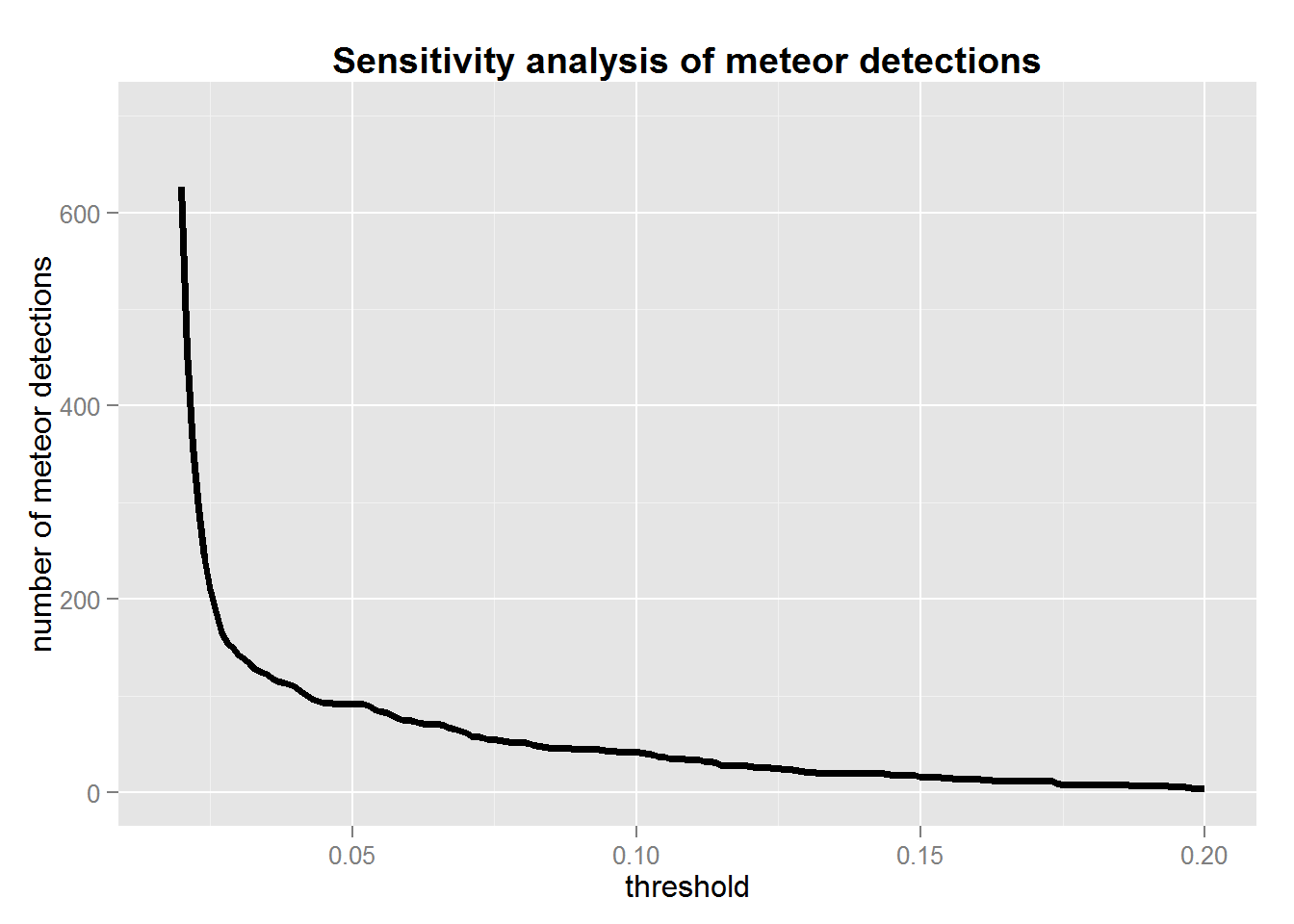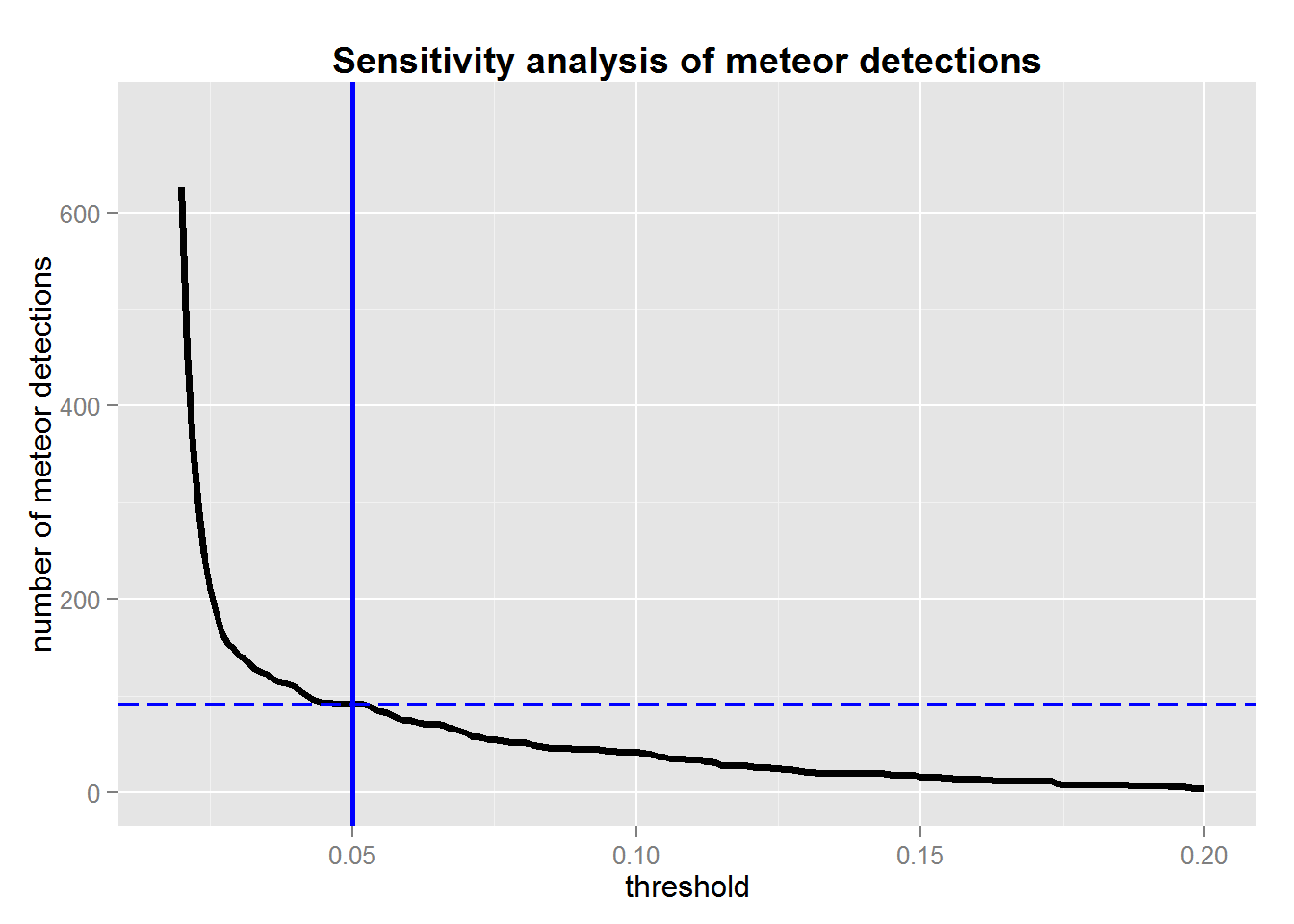www.astrostatistics.org/projects/meteors

In an earlier contribution I documented an implementation in R of the radio meteor detection algorithm proposed by Roelandts (2014). Applying the algorithm I found too many false positives: the algorithm finding meteors when there are none. The obvious thing to do is to increase the level of the threshold value in the algorithm. The question is to what level?

On this page I report on a sensitivity analysis I conducted looking at how meteor detection is dependent on the threshold value. This analysis results in a tentative approach to selecting an optimal threshold value.

## Varying the threshold parameter

The meteor detection function makes use of a number of parameters that can be adjusted. Most of the parameters relate to the specifics of the signal and signal processing such as the carrier frequency and the sampling frequency. There is one parameter, the threshold value, which plays a determinant role in the detection algorithm. The threshold is applied to the indicator signal and directly affects the number of meteor detections. Low threshold values result an many detections and high values in few.

I used data from the station at Uccle, BEUCCL, collected on 3 January 2015 – with the aim of studying the Quadrantids meteor shower in a next stage. For the sensitivity analysis I used the first four hours worth of recordings on that day, which amounts to 48 files covering 5 minutes of observations each. I varied the threshold level from 0.010 to 0.300 in steps of 0.001. Note that the deafult value of 0.025 falls in this range.

## Results

I applied the detection method to each of the files, with all threshold values under consideration, and looked at the total meteor count in these four hours. This plot shows the result:The threshold value is shown on the horizontal axis, and the meteor count on the vertical. As expected, the higher the threshold value the lower the number of detections. Threshold values of higher than 0.271 result in no detections at all in this data set.

A potential interpretation of the shape of the curve is this: low threshold values result in many false positives, i.e. detections of meteors that are in actual fact just noise. This is the case at the lefthand side of the plot. When the threshold value is increased, the number of detections in the noise decreases rapidly. At some stage there is an optimum where no detections occur in the noise but all true meteors are still present. Increasing the threshold level further, also the true meteor detections will disappear and the total count will gradually tend to zero.

The challenge is to pick that threshold level where no noise detections occur but where all true meteors are still detected. If the indicator signal is perfect such point exist. In reality, however, the separation between false and true detections is probably less well defined and a balance is sought minimizing the false detections and maximizing the true. I speculate that level to be at the point where the initially rapid decrease stabilizes and the sensitivity curve tends to a constant level (“flattens out”), before there is a gradual loss of true meteors. In the present data set, this would be at a threshold level of 0.050, as shown below. At this level, 92 meteors are detected (dashed line in plot below). In fact, all threshold levels in the range [0.047; 0.052] would lead to this number of detections. Using the default value of 0.025 for the threshold, 210 meteors would be detected.## Conclusion

The sensitivity analysis presented here studies the effect of the threshold parameter on the number of meteors detected by the method of Roelandts (2014). A tentative recommendation is to choose the threshold value at a point where the sensitivity curve levels out but before it starts a gradual decline to zero. It is conjectured that this avoids detections in the noise while at the same time retaining all true meteors. Obviously a perfect separation of true and false detections is unlikely to be possible, but this choice should strike an optimal balance.

I will use the threshold value of 0.050 in future research using data from the BEUCCL station. I recommend conducting such a sensitivity analysis for each station the data of which you want to analyze, and possibly reiterating this at different points in time.

Check here for more on meteor detections: www.astrostatistics.org/projects/meteors